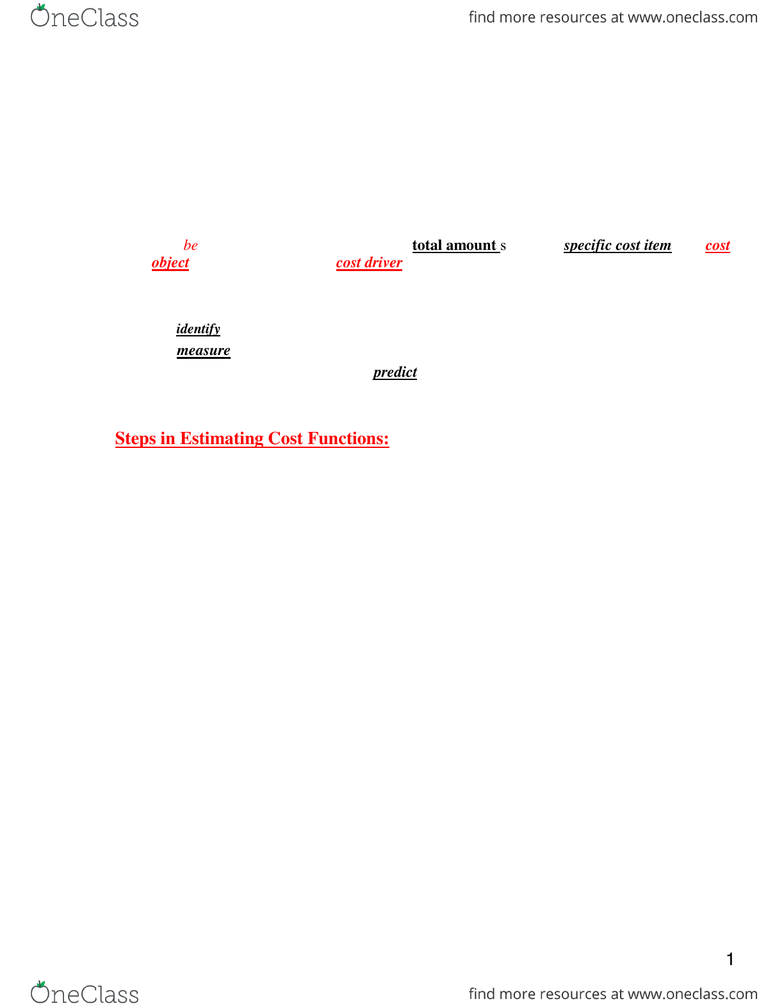Class Notes (1,100,000)
CA (620,000)
McMaster (50,000)
COMMERCE (2,000)
Lecture 10

# COMMERCE 2AB3 Lecture Notes - Lecture 10: Westjet, Fixed Cost, Cost Estimate

Department
Commerce
Course Code
COMMERCE 2AB3
Professor
Mohamed Shehata
Lecture
10

Page:
of 5Basic Cost concepts and terms
1
Chapter 10
Cost Estimation
Cost estimation is process of developing a well-defined functional (i.e., Mathematical) expression
that explain the relationship between a cost object and its cost driver(s) in order to understand its
cost behavior pattern.
Cost behaviour describes the way that the total amount spent of a specific cost item on a cost
object reacts to changes in its cost driver(s) (e.g., production quantity or sales volume).
There three possible cost behavior: variable, Fixed and Mixed.
The purposes of cost estimation are:
(1) to identify the key cost drivers for each cost object to help managers control costs
(2) to measure the variable and/or fixed components of the total cost, and
(3) to use the estimated parameters to predict its value in the future so as to help managers
correctly plan for the future.
Steps in Estimating Cost Functions:
There are six steps in the cost estimation process:
1. Define the Cost item (object) to be estimated.
2. Determine the Cost Driver(s).
3. Collect Consistent and Accurate historical Data about the past behavior.
4. Plot (Graph) the Data.
5. Select and Employ the Estimation Method.
6. Assess the Accuracy of the Cost Estimation.
METHODS OF COST ESTIMATION
There are two major methods commonly used in estimating cost functions:
1. The high-low method considers only two points of data, the highest and lowest, for
activity within the relevant range.
2. The least-squares regression method is a statistical approach that is both objective
and considers all data points.
What is a cost function?
It is a mathematical expression describing how the total costs of a cost item (object) change with
changes in the level of a related cost driver(s).
Basic assumptions
(1) Changes in total costs can be explained by changes in the level of a single cost driver.
(2) Cost behavior can adequately be approximated by a linear function of the activity level within the
relevant range
Basic Cost concepts and terms
2
Based on the above two assumptions, we can express the relationship between a particular cost item
and its cost driver in a mathematical form using the straight line equation as follows:
y = a + b(X)
where: y = Total costs
a = fixed cost component
b = slope coefficient (variable cost rate)
X = the volume (quantity) of the cost driver
Note that:
1. If the estimated value of a = 0 and b > 0 then we can conclude that the cost behavior
isVariable.
2. If the estimated value of b = 0 and a > 0 then we can conclude that the cost behavior is
Fixed.
3. If the estimated values of both a & b > 0 then we can conclude that the cost behavior is Mixed.
Example:
Holiday Inn Hotel in Toronto offers WestJet airline three alternative cost structures to accommodate
its crew overnight:
1. \$60 per night per room usage:
y = \$60x
The slope of the cost function is \$60
2. \$8,000 per month
y = \$8,000
\$8,000 is called a constant or intercept.
The slope of the cost function is Zero
3. \$3,000 per month plus \$24 per room
y = \$3,000 + \$24x
Basic Cost concepts and terms
3
High-Low (HL) Method
1. Identify the highest value of volume (X) and its associated value of cost (Y)
2. Identify the Lowest value of volume (X) and its associated value of cost (Y)
3. Use the above two points to estimate the parameter b (variable cost per unit) in the following
cost function:
b = Change in cost (y) (Hy Ly)
Change in volume (x) (Hx Lx)
4. Estimate the Fixed component a as follows:
Substitute the value of b into the basic equation along with actual values of Y and X at either the highest
point or the lowest point into the following equation; then solve for the intercept a:
y = a + b(x)
Total Cost (lowest cost driver level) = a + b(x at lowest quantity of cost driver)
Using the highest cost-quantity pair will give the same answer for the intercept.
5. Use the estimated cost function to predict future costs.